Kinematics Exam2 and Problem Solutions

Kinematics Exam2 and  Problem Solutions

1. An object is dropped from 320 m high. Find the time of motion and velocity when it hits the ground.(g=10m/s2)

h=1/2.g.t2  , v=g.t

h=320m

g=10m/s2

320=1/2.10.t2

t=8s.

v=g.t=10.8=80m/s

2. An object does free fall and it takes 60m distance during last 2 seconds of its motion. Find the height it is dropped.(g=10m/s2)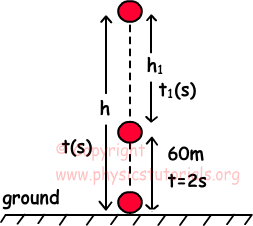t is the time of motion

h=1/2.g.t2

h1=1/2.g.t12

put t1=t-2 and h-h1=60 in the equation,

1/2.g.t2-1/2.g.t12=60

5t2-5(t2-4t+4)=60

t=4s

h=1/2.g.t2=1/2.10.42=80m

3. An object is dropped from 144m height and it does free fall motion. Distance it travels and time of motion are given in the picture below. Find the distance between points B-C.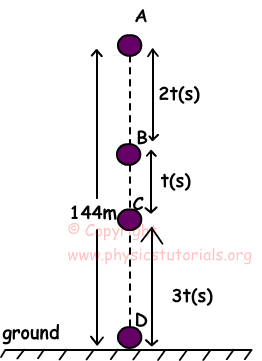We can draw velocity time graph of object and area under this graph gives us position of the object.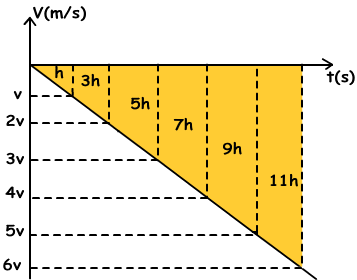As you can see from the velocity time graph, object travels 5h distance during 2t-3t which is the distance between the points B and C.

All distance traveled is 36h

144m=36h

h=4m

Distance between B-C=5h=5.4m=20m

4. A hot- air balloon having initial velocity v0 rises. Stone dropped from this balloon, when it is 135 m height, hits the ground after 9 s. Find the velocity of the balloon.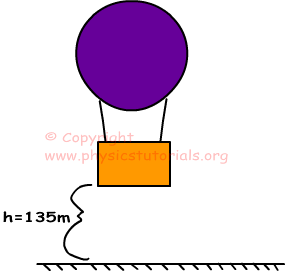-h=v0.tfligth-1/2.g.tfligth2

-135=v0.9-1/2.10.(9)2

-135=9vo-405

9v0=270

v0=30m/s

5. Look at the given picture below. Object K does free fall motion and object B thrown upward at the same time. They collide after 2s. Find the initial velocity of object B. (g=10m/s2)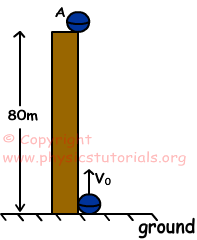Object A does free fall motion

hA=1/2.10.22=20m

hL=v0.t-1/2.g.t2

hL=v0.2-1/2.10.22

hL=2v0-20

hK+hL=80m

20m+hL=80m

2v0-20=60m

v0=40m/s

Author:

Tags: